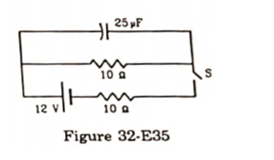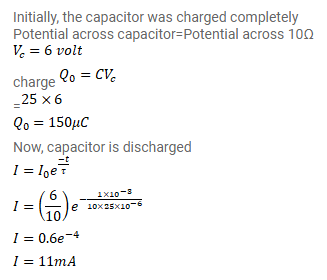# The Switch S shown in figure (32-E35) is kept closed for

Question:

The Switch S shown in figure (32-E35) is kept closed for a long time and is then opened at $t=0$

Find the current in the middle $10 \Omega$ resistor at $t=1.0 \mathrm{~ms}$Solution: